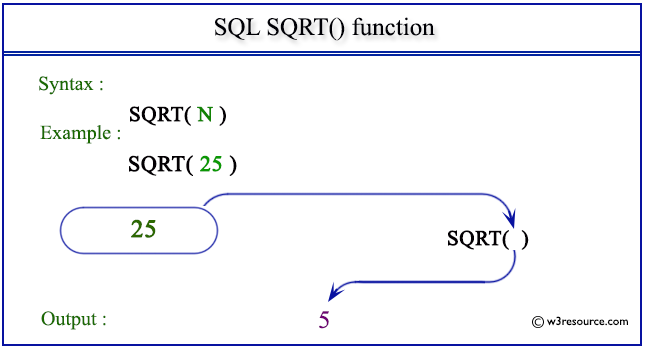# SQL SQRT() function

## SQRT() function

SQL SQRT() returns the square root of a given value in the argument.

Syntax:

`SQRT( expression )`

Parameters:

Name Description
expression An expression which is a numeric value or numeric data type.

MySQL, PostgreSQL, SQL Server, and Oracle

All of above platforms support the SQL syntax of SQRT().

## SQL SQRT() function: Pictorial presentationExample :

To get the square root of 36 from the DUAL table, the following SQL statement can be used:

``````SELECT SQRT(36)
FROM dual;
``````

Output:

```  SQRT(36)
----------
6
```

## SQL SQRT() function with distinct

Sample table: customer

To get the unique square root of 'opening_amt' from 'customer' table, the following SQL statement can be used :

``````SELECT SQRT(opening_amt)
FROM customer;
``````

Output:

```SQRT(OPENING_AMT)
-----------------
77.4596669
54.7722558
70.7106781
70.7106781
63.2455532
77.4596669
70.7106781
83.6660027
83.6660027
89.4427191
83.6660027
83.6660027
83.6660027
70.7106781
63.2455532
100
77.4596669
89.4427191
70.7106781
83.6660027
89.4427191
89.4427191
89.4427191
89.4427191
83.6660027
```

Note: Outputs of the said SQL statement shown here is taken by using Oracle Database 10g Express Edition.

Here is a slide presentation which covers the SQL arithmetic functions.

Practice SQL Exercises